One of the earliest indicators of a degradation of the EPHIN detectors is a decrease in the resolution of the detectors. To track this requires analysis of the PHA buffer data, which stores the pulse-height information from individual coincidence events. The PHA buffer contains a sequence of variable length records, where each record stores information on the coincidence type (e.g. E150), direction of travel via which detector A and B segments are triggered, pulse-heights of the detectors that triggered, and high/low gain selection on the pulse-heights. These data, when combined with supplied calibrations (Mueller-Mellin 2000), can be converted to particle energy-loss in the individual EPHIN detectors (A through E).

Figure 1 is a scatter plot of the energy loss in the A detector versus the summed energy loss in detectors A through E for all coincidences. The energy loss in detector A approximates the dE/dx energy loss by the particle per unit length in traveling through matter. dE/dx is proportional to (Z/v)2, where Z is the particle charge and v is particle velocity. The total energy loss of a particle stopped in the EPHIN is the particle kinetic energy (1/2mv2). Thus the product (dE/dx)×E is proportional to mZ2 so that different isotopes should roughly follow lines with a slope of -1 in figure 1. The solid line is a calibration curve that represents the expected location of alpha particles (4He), after accounting for a break-down in the assumption that the energy loss in detector A is a good approximation for dE/dx. The dark band that parallels it at lower total energy loss is due to protons. Much of the band of points that crosses the proton and alpha particle bands is due to particles that are not stopped within detectors A-E, the Integral channel, and so the summed energy losses does not reflect the particle kinetic energy.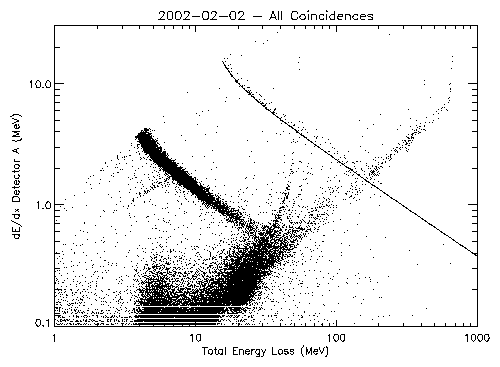Figure 1: Energy loss in Detector A versus the energy loss in detectors A through E. All coincidences are included in the event selection. Coincidences with the lowest total and detector A losses are not shown (off plotted scale). The alpha particle calibration is shown as a solid line.

The data presented in figure 1 can be cleaned up via filtering. We can reject coincidences that are identified as being from the Integral channel and those whose directions are from large angles relative to the EPHIN axis. Figure 2 is a plot similar to figure 1 where we have made this discrimination. Note that the events that cross the proton and alpha bands have been removed.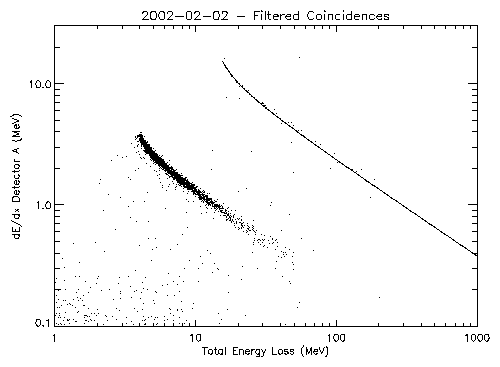Figure 2: Energy loss in Detector A versus the energy loss in detectors A through E. Integral channel coincidences are not included. The coincidences were also required to pass through the same segments on the A and B detectors to restrict the acceptance angle.

The width of the proton band depends on the detector resolution. The events in figure 2 can be "rotated" into a space where the distance of a point from the 4He calibration curve can be calculated. A histogram of these distances shows peaks from the different particles as shown in figure 3.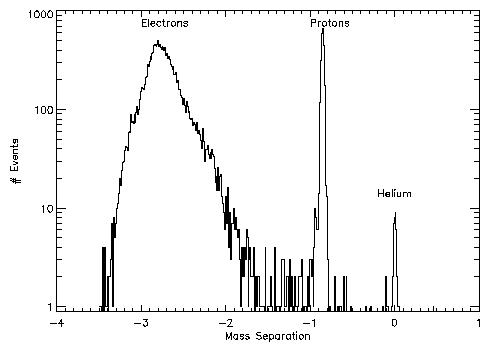Figure 3: Histogram of mass separations from the Helium calibration curve.

We can concentrate on just the proton peak and do a comparison of data from early in the mission (1999-Aug-25) to more recent data (2002-Feb-02). These data are shown in figure 4. The width of the recent peak is not noticeably wider than the one from early in the mission suggesting that there has not been significant degradation in the EPHIN detectors. We should begin a long-term process of monitoring the width of this peak as a function of time for signs of broadening of this peak. It may be necessary to filter out times when we are in the radiation zones or possibly times affected by solar events.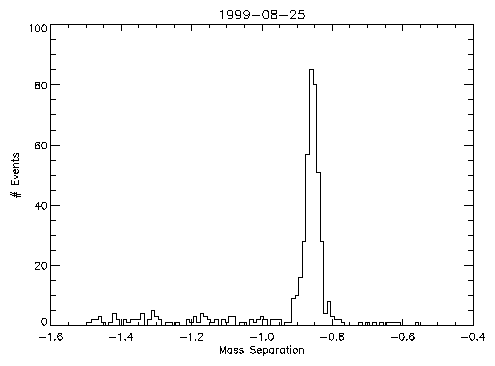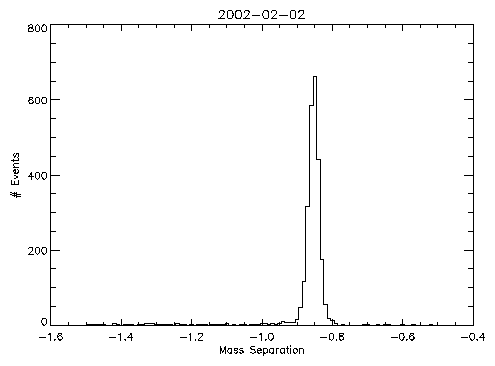Figure 4: Proton peaks from mass separation histograms. Top) data from 1999-Aug-25 Bottom) data from 2002-Feb-02.
As an initial attempt at looking for any trends with time, I have divided the EPHIN data into orbits, selecting times on each orbit when CHANDRA was at geocentric distance greater than 80,000 km. For each orbit interval I have selected the events with a mass separation in the range -0.9 to -0.8 (i.e. the proton peak) and calculated the mean and RMS deviation from the mean. The results are plotted in figure 5. Some of the large upward fluctuations in width are due to a limited number of events in the peak, while others are associated with times of high solar activity (e.g. July 2000 or Nov 2000). The width seems to have a "floor" that may have risen very slightly with time. A possibly more interesting trend is the change in the mean with time. This change may be due to calibration changes caused by the temperature increase or by detector degradation.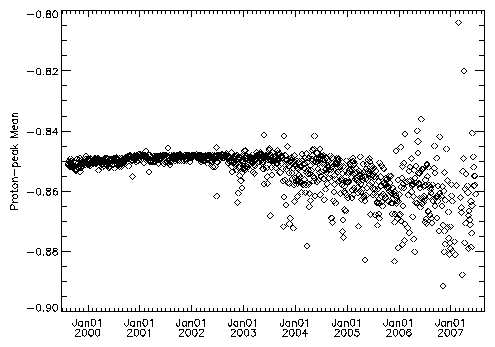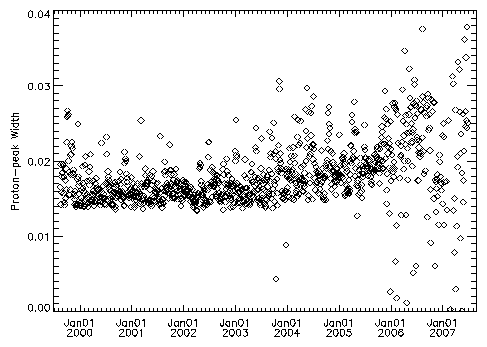Figure 5: Proton-peak Mean (top) and width (bottom) versus time.

Tabulation of the data for individual orbit intervals can be veiwed on this page.

## References

"Correction of CHANDRA EPHIN Counting Rate Measurements", Reinhold Mueller-Mellin, 2000, memo.